NCERT Solutions for Class 2 Maths Chapter 2 Counting in Groups are part of NCERT Solutions for Class 2 Maths. Here we have given NCERT Solutions for Class 2 Maths Chapter 2 Counting in Groups.

 Board CBSE Textbook NCERT Class Class 2 Subject Maths Chapter Chapter 2 Chapter Name Counting in Groups Number of Questions 12 Category NCERT Solutions

## NCERT Solutions for Class 2 Maths Chapter 2 Counting in Groups

NCERT Textbook Pages 9-10
Look and Guess the Number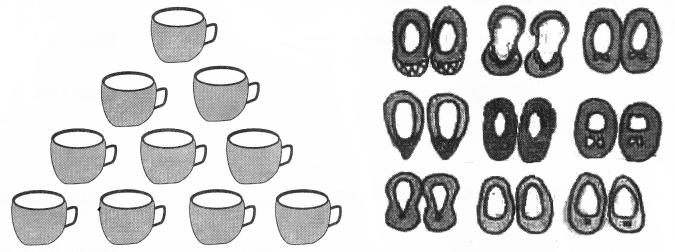Question 1.
Can you guess how many cups?
3 and 3 is 6 cups and 4 cups below. That should be 10 cups.

Question 2.
Can you guess how many pairs of shoes?
There are 3 lines with 3 pairs of shoes in each line. That makes 9 pairs of shoes.

Question 3.
Look at how different things are kept in groups. Try to guess the total number without counting each thing.(a) 12 glasses
(b) 25 bangles
(c) 12 pairs of earrings

Question 4.
There are three groups of spoons.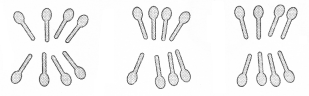(a) How many in each group?
(b) Guess the total number of spoons.
(a) 8.
(b) 24.

NCERT Textbook Page 11
More or Less, Let Us Guess
Question 5.
(a) Number of teeth in your mouth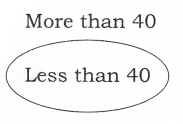(b) Number of seeds in an orange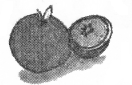(c) Number of mathsticks in a matchbox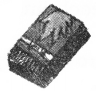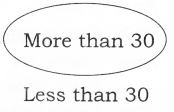(d) Number of pencils in your class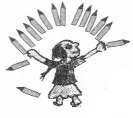(e) Number of spokes in one cycle wheel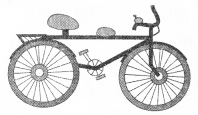NCERT Textbook Page 12
Hop till You Drop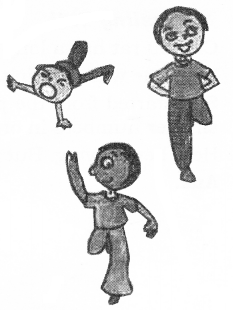Question 6.
How many times can you hop on your right foot without falling?
6 times

Question 7.
How many times can you hop on your left foot without falling?
5 times

Join the Dots
Question 8.
Jojo doggy is hungry. Join the dots in order, from 21 to 52, and find out what is hidden for him to eat.
Bone.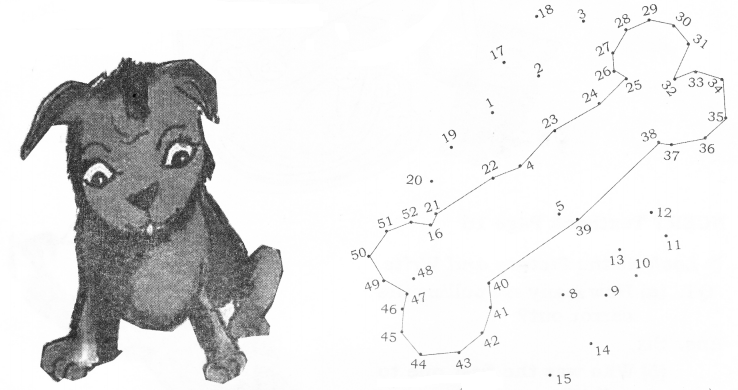NCERT Textbook Page 13
Naughty Bhurru
Bhurru has torn some pages of this book.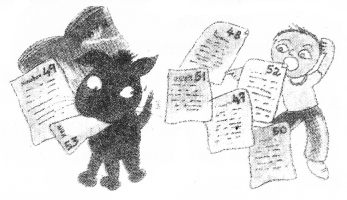Question 9.
Write the page numbers in the correct order.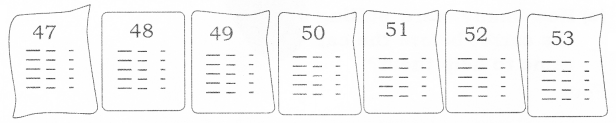Puzzling Tail
10. Chipku rat has a long tail.
When he was sleeping, naughty cat thought of tying his tail to the poles. She started from the pole with the biggest number. She moved on to the
smaller numbers in order.
Help her in tying. But the tail should not cross itself anywhere.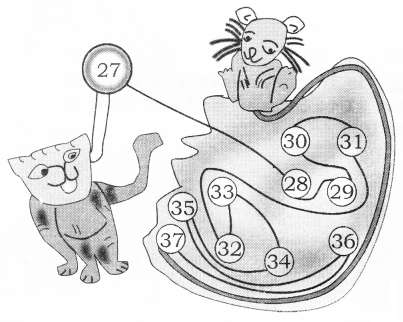NCERT Textbook Page 16
Look at the Picture and Write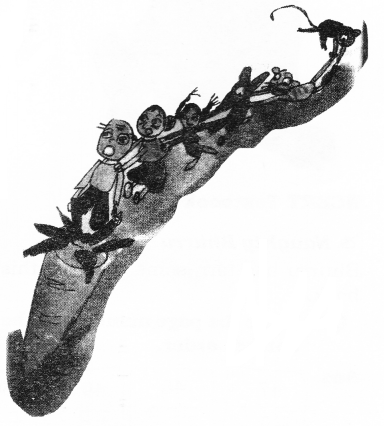Question 11.
(a) How many are pulling the carrot out?
(b) Who was the first one to pull It?
(c) The cat Is in the line.
(d) Who was the fourth one to pull the carrot?
(e) How many were pulling the carrot before the cat came to help them?
(f) Make a cap on the third one In the line.
(g) Make a moustache for the sixth one in the line.

(a) Six.
(b) The old man.
(c) Fifth.
(d) The dog.
(e) Four.
(f) Do it yourself.
(g) Do it yourself.

NCERT Textbook Page 17
Seema’s Century
Seema has made a design with different bindis.Question 12.
(a) Look at the groups and guess the total number of bind is.
(b) Draw more groups to coffiplete 100 bindis. How many more bindis did you have to draw?